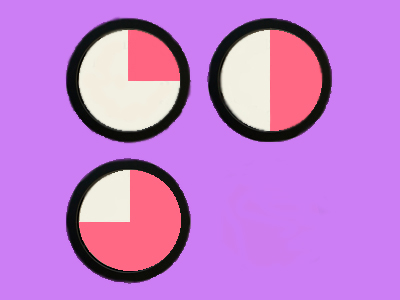14,  12,  34... what comes next in this sequence?

# Year 2 Fractions - Counting Up to 10

This quiz addresses the requirements of the National Curriculum KS1 Maths and Numeracy for children aged 6 and 7 in year 2. Specifically this quiz is aimed at the section dealing with counting in fractions up to 10.

Being able to count up in fractions means understanding the value of a fraction and recognising how many of each makes a whole. For example, when counting up in quarters, it will take four steps to reach one whole and 40 to reach 10. Children in Year 2 are expected to be able to count up to ten in fractions and this quiz will help them.

Question 1
Which three numbers come next?
312, 334, 4
4, 5, 6
434, 5, 534
424, 434, 4 44
414, 412, 434
The numbers are one quarter more each time
Question 2
What is wrong with this sequence:
314, 324, 312, 334
334 is too large for the sequence
314 is in the wrong place
324 is the same as 312
There aren't enough numbers in the sequence
324 has exactly the same value as 312 so shouldn't be there!
Question 3
What comes next: 24, 34, 1
44
114
2 14
1 34
Counting up in quarters means that after 1, it will be 1 + 14 more
Question 4
Which two numbers are missing:
3, ___, 312, 334, 4, ___
324 and 412
314 and 4
414 and 514
314 and 414
Each number is one quarter more than the one before it
Question 5
Which two numbers are missing:
512, ___, 612, ___
6 and 7
5 and 6
7 and 8
6 and 9
The sequence is going up in halves
Question 6
What is happening in this sequence:
214, 224, 234, 3
The numbers increase by 24 each time
The numbers increase by 12 each time
The numbers increase by 13 each time
The numbers increase by 14 each time
Each number is 14 more than the one before it
Question 7
Which two numbers are next in this sequence?
214, 212, 234
3, 312
334, 4
244, 3
3, 314
Adding a quarter to 234 makes 3, and then adding another quarter makes 314
Question 8
What comes next: 1, 112, 2, 212
2
312
3
222
Adding another half to 212 results in 3
Question 9
Which two numbers come next in this sequence?
412, 5, 512, 6
522, 6
612, 7
6, 7
614, 7
The sequence is going up by 12 each time
Question 10
What are the next three numbers in this sequence?
7, 714, 712
734, 744, 754
734, 8, 814
7, 812, 9
744, 8, 9
The sequence is going up by a quarter each time. 712 is the same as 724